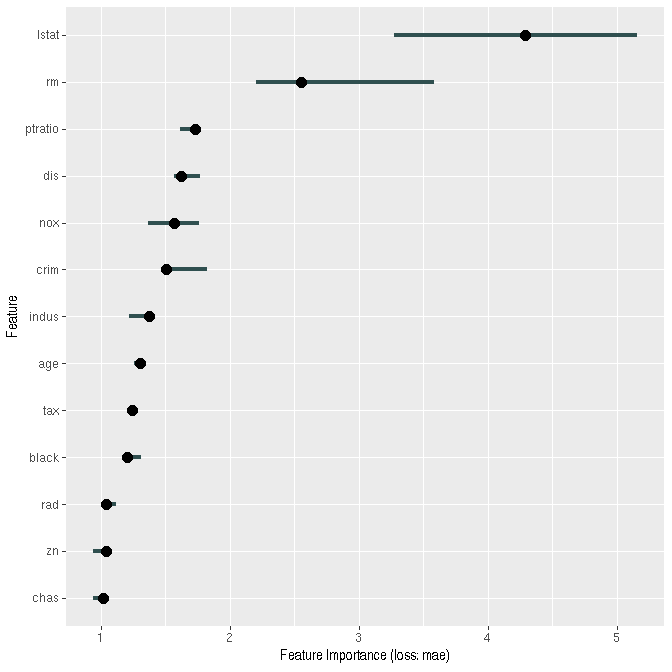# Parallel computation of interpretation methods

The iml package can now handle bigger datasets. Earlier problems with exploding memory have been fixed for FeatureEffect, FeatureImp and Interaction. It’s also possible now to compute FeatureImp and Interaction in parallel. This document describes how.

First we load some data, fit a random forest and create a Predictor object.

set.seed(42)
library("iml")
library("randomForest")
data("Boston", package  = "MASS")
rf = randomForest(medv ~ ., data = Boston, ntree = 10)
X = Boston[which(names(Boston) != "medv")]
predictor = Predictor$new(rf, data = X, y = Boston$medv)

## Going parallel

You need to install the doParallel or a similar framework to compute in parallel. Before you can use parallelization to compute for example the feature importance on multiple CPU cores, you have to setup up a cluster. Fortunately, the doParallel makes it easy to setup and register a cluster:

library("doParallel")
# Creates a cluster with 2 cores
cl = makePSOCKcluster(2)
# Registers cluster
registerDoParallel(cl)

Now we can easily compute feature importance in parallel. This means that the computation per feature is distributed among the 2 cores I specified earlier.

imp = FeatureImp$new(predictor, loss = "mae", parallel = TRUE) plot(imp)That wasn’t very impressive, let’s actually see how much speed up we get by parallelization. system.time(FeatureImp$new(predictor, loss = "mae", parallel = FALSE))
#>    user  system elapsed
#>   1.300   0.008   0.342
system.time(FeatureImp$new(predictor, loss = "mae", parallel = TRUE)) #> user system elapsed #> 0.096 0.000 1.767 A little bit of improvement, but not too impressive. Parallelization is more useful in the case where the model uses a lot of features or where the feature importance computation is repeated more often to get more stable results. system.time(FeatureImp$new(predictor, loss = "mae", parallel = FALSE, n.repetitions = 20))
#>    user  system elapsed
#>   4.080   0.004   1.053
system.time(FeatureImp$new(predictor, loss = "mae", parallel = TRUE, n.repetitions = 20)) #> user system elapsed #> 0.096 0.000 11.664 Here the parallel computation is twice as fast as the sequential computation of the feature importance. The parallization also speeds up the computation of the interaction statistics: system.time(Interaction$new(predictor, parallel = FALSE))
#>    user  system elapsed
#>  10.740   0.020   3.681
system.time(Interaction$new(predictor, parallel = TRUE)) #> user system elapsed #> 0.068 0.000 112.688 Same for FeatureEffects: system.time(FeatureEffects$new(predictor, parallel = FALSE))
#>    user  system elapsed
#>   0.812   0.000   0.210
system.time(FeatureEffects\$new(predictor, parallel = TRUE))
#>    user  system elapsed
#>   0.048   0.008   4.164

Remember to stop the cluster in the end again.

stopCluster(cl)